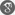##### Cite as:
B.C. Dhage, G.T. Khurape, A.Y. Shete, J.N. Salunkhe, Existence and Approximate Solutions for Nonlinear Hybrid Fractional Integrodifferential Equations, Int. J. Anal. Appl., 11 (2) (2016), 157-167.

#### Abstract

In this paper we prove existence and approximation of the solutions for initial value problems of nonlinear hybrid fractional differential equations with maxima and with a linear as well as quadratic perturbation of second type. The main results rely on Dhage iteration method embodied in the recent hybrid fixed point theorem of Dhage (2014) in a partially ordered normed linear space. The approximation of the solutions of the considered nonlinear fractional differential equations are obtained under weaker mixed partial continuity and Lipschitz conditions. Our hypotheses and the main results are also illustrated by a numerical example.

#### References

1. M. Ammi, E. El Kinani, D. Torres, Existence and uniqueness of solutions to functional integro-differential fractional equations, Electron. J. Diff. Equ., 2012 (2012), Art. ID 103.2. M. El Borai, M. Abbas, On some integro-differential equations of fractional orders involving Carathéodory nonlinearities, Int. J. Mod. Math. 2 (2007), 41-52.3. B.C. Dhage, Fixed point theorems in ordered Banach algebras and applications, PanAmer. Math. J. 9(4) (1999), 93–102.4. B.C. Dhage, Basic results in the theory of hybrid differential equations with mixed perturbations of second type, Funct. Diff. Equ. 19 (2012), 1-20.5. B.C. Dhage, Quadratic perturbations of periodic boundary value problems of second order ordinary differential equations, Differ. Equ. Appl. 2 (2010), 465–486.6. B.C. Dhage, Hybrid fixed point theory in partially ordered normed linear spaces and applications to fractional integral equations, Differ. Equ. Appl. 5 (2013), 155-184.7. B.C. Dhage, Partially condensing mappings in partially ordered normed linear spaces and applications to functional integral equations, Tamkang J. Math. 45(4) (2014), 397-426.8. B.C. Dhage, Nonlinear D-set-contraction mappings in partially ordered normed linear spaces and applications to functional hybrid integral equations, Malaya J. Mat. 3(1)(2015), 62-85.9. B.C. Dhage, Operator theoretic techniques in the theory of nonlinear hybrid differential equations, Nonlinear Anal. Forum 20 (2015), 15-31.10. B.C. Dhage, A new monotone iteration principle in the theory of nonlinear fractional differential equations, Intern. J. Anal. Appl. 8(2) (2015), 130-143.11. B.C. Dhage, S.B. Dhage, Approximating solutions of nonlinear first order ordinary differential equations, Glob. J. Math. Sci. 2 (2014), 25-35.12. B.C. Dhage, S.B. Dhage, S.K. Ntouyas, Existence and approximate solutions for fractional differential equations with nonlocal conditions, Journal of Fractional Calculus and Applications 7(1) (2016), 24-35.13. B.C. Dhage, S.B. Dhage, S.K. Ntouyas, Dhage iteration method for existence and approximate solutions of nonlinear quadratic fractional differential equations, Journal of Fractional Calculus and Applications, 7(2) (2016), 132-144.14. B.C. Dhage, S.K. Ntouyas, Existence results for boundary value problems for fractional hybrid differential inclucions, Topol. Methods Nonlinear Anal. 44 (2014), 229-238.15. S. Heikkilä and V. Lakshmikantham, Monotone Iterative Techniques for Discontinuous Nonlinear Differential Equations, Marcel Dekker inc., New York 1994.16. A.A. Kilbas, H.M. Srivastava, J.J. Trujillo, Theory and Applications of Fractional Differential Equations, North-Holland Mathematics Studies, 204. Elsevier Science B.V., Amsterdam, 2006.17. I. Podlubny, Fractional Differential Equations, Academic Press, San Diego, 1999.18. S. Sun, Y. Zhao, Z. Han, Y. Li, The existence of solutions for boundary value problem of fractional hybrid differential equations, Commun. Nonlinear Sci. Numer. Simul., 17 (2012), 4961-4967.19. Y. Zhao, S. Sun, Z. Han, Q. Li, Theory of fractional hybrid differential equations, Comput. Math. Appl. 62 (2011), 1312-1324.